# CAT-2021-QA-Slot-1/Free CAT Question papers

1).

Amal purchases some pens at ₹ 8 each. To sell these, he hires an employee at a fixed wage. He sells 100 of these pens at ₹ 12 each. If the remaining pens are sold at ₹ 11 each, then he makes a net profit of ₹ 300, while he makes a net loss of ₹ 300 if the remaining pens are sold at ₹ 9 each. The wage of the employee, in INR, is

2).

The strength of an indigo solution in percentage is equal to the amount of indigo in grams per 100 cc of water. Two 800 cc bottles are filled with indigo solutions of strengths 33% and 17%, respectively. A part of the solution from the first bottle is thrown away and replaced by an equal volume of the solution from the second bottle. If the strength of the indigo solution in the first bottle has now changed to 21% then the volume, in cc, of the solution left in the second bottle is:

3).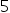-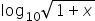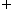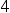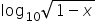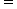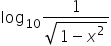, then 100x equals

4).

f(x) =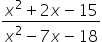is negative if and only if

1. -5 < x < -2 or 3 < x < 9

2. -2 < x < 3 or x > 9

3. x < -5 or 3 < x < 9

4. x < -5 or -2 < x < 3

5).

The number of integers n that satisfy the inequality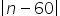<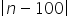<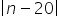is

1. 21

2. 18

3. 20

4. 19

6).

Identical chocolate pieces are sold in boxes of two sizes, small and large. The large box is sold for twice the price of the small box. If the selling price per gram of chocolate in the large box is 12% less than that in the small box, then the percentage by which the weight of chocolate in the large box exceeds that in the small box is nearest to

1. 127

2. 135

3. 124

4. 144

7).

If the area of a regular hexagon is equal to the area of an equilateral triangle of side 12 cm, then the length in cm of each side of the hexagon is equal to

1. 4√6

2. 6√6

3. 2√6

4. √6

8).

Onion is sold for 5 consecutive months at the rate of Rs. 10, 20, 25, 25, and 50 per kg, respectively. A family spends a fixed amount of money on onion for each of the first three months, and then spends half that amount on onion for each of the next two months. The averageexpense for onion, in rupees per kg, for the family over these 5 months is closest to

1. 18

2. 26

3. 16

4. 20

9).

If r is a constant such that has exactly three distinct real roots, then the value of r is

1. 15

2. 18

3. 17

4. 21

10).

Anil invests some money at a fixed rate of interest, compounded annually. If the interests accrued during the second and third year are ₹ 806.25 and ₹ 866.72, respectively, the interest accrued, in INR, during the fourth year is nearest to

1. Rs 931.72

2. Rs 926.84

3. Rs 929.48

4. Rs 934.65